# Markov chain, class of zero states of a

(diff) ← Older revision | Latest revision (diff) | Newer revision → (diff)

A setof states of a homogeneous Markov chainwith state spacesuch thatfor any,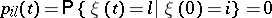for any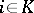,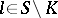,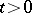, and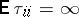(*)

for any, where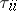is the return time to the state: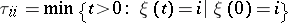for a discrete-time Markov chain, andfor a continuous-time Markov chain.

As in the case of a class of positive states (in the definition of a positive class (*) is replaced by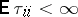), states belonging to the same zero class have a number of common properties. For example, for any states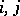of a zero class,An example of a Markov chain whose states form a single zero class is the symmetric random walk on the integers: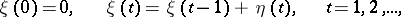whereare independent random variables,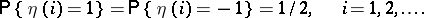How to Cite This Entry:
Markov chain, class of zero states of a. Encyclopedia of Mathematics. URL: http://encyclopediaofmath.org/index.php?title=Markov_chain,_class_of_zero_states_of_a&oldid=18811
This article was adapted from an original article by A.M. Zubkov (originator), which appeared in Encyclopedia of Mathematics - ISBN 1402006098. See original article